# Solving Problems with Polynomials Chapter Exam

Exam Instructions:

Choose your answers to the questions and click 'Next' to see the next set of questions. You can skip questions if you would like and come back to them later with the yellow "Go To First Skipped Question" button. When you have completed the practice exam, a green submit button will appear. Click it to see your results. Good luck!

### Page 1

#### Question 3 3. Subtract the following rational expressions.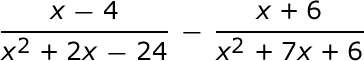#### Question 4 4. Add the following rational expressions.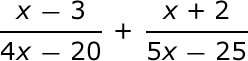#### Question 5 5. Add and simplify.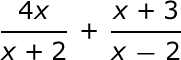### Page 2

#### Question 6 6. Subtract and simplify.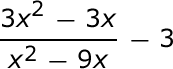### Page 3

#### Question 13 13. The graph of the function f(x) = x^3 - 4x^2 - 2x + 8 is shown below. This function has only one rational zero. Use the rational zeros theorem and the graph to determine the value of the rational zero.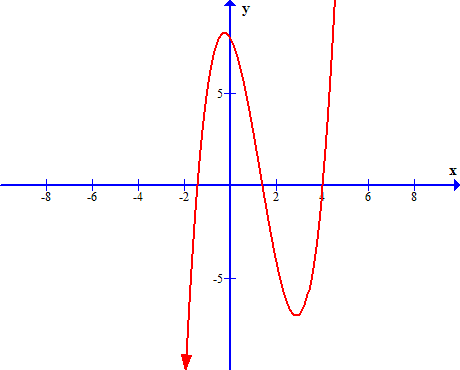#### Question 14 14. Which answer choice is not a possible rational zero for the following function?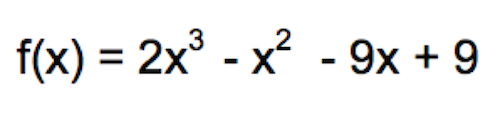### Page 4

#### Question 17 17. Solve the equation.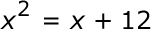#### Question 18 18. Find the roots of the equation.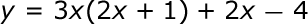### Page 6

#### Question 26 26. Add the following rational expressions.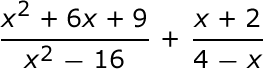#### Question 27 27. Add and simplify.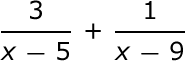#### Solving Problems with Polynomials Chapter Exam Instructions

Choose your answers to the questions and click 'Next' to see the next set of questions. You can skip questions if you would like and come back to them later with the yellow "Go To First Skipped Question" button. When you have completed the practice exam, a green submit button will appear. Click it to see your results. Good luck!

Support